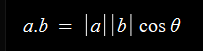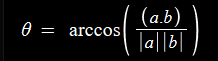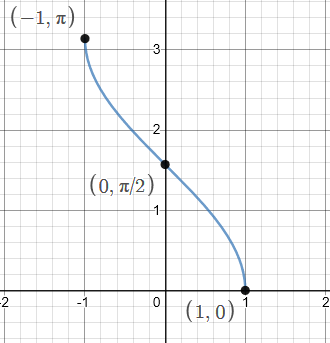# The Maths

The math needed for this is basic knowledge of trigonometric functions and of dot products, which you can find here and here respectively.

with that said, lets dive in!

## The circle

above image represents the unit circle

the text indicates the rotation in radians at each point, for those of you unfamiliar with radians, pi radians is 180 degrees.

This shows the rotations counterclockwise, you should also know that rotations that are clockwise are considered negative and can be expressed as 2π + θ (or 2π - |θ|), due to the nature of circles(θ being the variable most commonly used for unknown angles).

Also important (marked with red line) is the length associated with the cosine value for each rotation. You can see from the diagram that cosine is an even function, meaning for any given θ, `cos(-θ) = -cos(θ)`, this will be important for later.

Since the equation for the dot product is:We can use this, and inverse trig functions to solve for theta:it is useful to note that arccosine has a domain of [-1,1], due to the periodic nature of cosineDue to this, and that from a bird’s eye view, a 0 degree rotation would face towards the top, rather than the right, a diagram of rotations from the front , instead of a traditional circle, would look something like this:

# Application

With the principle said, lets get onto applying this in game.

## Constants

In order to achieve the desired goals, we must first establish some constant values, namely

• Field / Range / Limit (max rotation on either side)
• Maximum Distance (optional) (maximum distance where a target can be seen)
• origin (what the object is “looking” from)

I will be going with 45 degrees for the first, 45 studs for the 2nd , and the head of the objects for the 3rd

## Getting the relative vector

getting the relative vector from point A to point B is the vector from the origin to point B minus the vector from the origin to point A, or `A -> B = B-A`, which can be seen from the following:

we can then get the directional component of this vector by using `[vector].Unit`

to find our forward facing vector, we can just use the lookvector of the object we are using

note that since both of these vectors are unit vector, we don’t need to explicitly divide by their magnitudes, allowing us to simply take the arccosine of the value given by v1:Dot(v2)

## Putting it all together

finally, we are at the stage where we can assemble everything, but first, the steps

1. define constants
2. go through all targets
3. establish forward vector,other vector, and angle between vectors
4. check if they and in the field of view and within maximum distance
5. do whatever you want

which would look something like this:

hidden code, for if you wish to try yourself
``````--step 1
local defaultColor = BrickColor.new("Medium stone grey")
local maxDist = 45
local range = 45
local targets = [Target]

--step 2
for _,target in ipairs(targets:GetChildren()) do
target.Changed:Connect(function()
--step 3
local side = relative.Unit
local theta = math.deg(math.acos(forward:Dot(side)))
--step 4
if (relative.Magnitude < maxDist) and (theta <= range) then
--step 5
target.BrickColor = BrickColor.new("Lime green")
else
target.BrickColor = defaultColor
end
end)
end
``````

## Result

With that done , the finished result should look something like this:
https://gyazo.com/0db81873bf09fb6ef7b289af4f3d1d39

with the added effect of having a conical field:
https://gyazo.com/c8425a41a2a49edb5a3d5b467f6f7bc1

This method also is able to be used for many different angles, as seen here
https://gyazo.com/5f11fd6b595d973bfe43fb5a73708eea

59 Likes

I have no idea how the Math works honestly, what is the use case for this?

NPCs FOV?

Since there are better ways for checking player’s FOV using the Camera unless in first person? I’m not entirely sure.

5 Likes

Exploit detection in FPS games most likely, allows the server to see if the part is actually able to be shot, etc

2 Likes

Arghhh… too much math here. But good tutorial though. I would appreciate if you could explain all this to a 7th grader.

3 Likes

Hey, this tutorial seemed pretty nice since field of sight is commonly seen as a good reason to learn dot products for games.

As feedback, I think you should have added the visualization for dot products as well in correlation to the tutorial but I’m not very good at math so it might just be me.

2 Likes

very informative tutorial!, and I agree with ArtFoundation some better visualizations would make this easier to understand, and you could probably simplify that math a little more. (explanation wise)

now i’m not really sure what this would be used for exactly other then NPCs, but I can already see other use cases with this sort of math, not just FOS.

still a wonderful tutorial nonetheless.

2 Likes

Thanks!

Although I understand the reason one would want more visuals, I myself prefer learn with visual representations vs lectures on most things, but the main reasons I didn’t add more visualizations was I didn’t want to add more “bloat” to it, with there already being 4 pretty big images and 3 gifs, and that I thought the link I provided for it explained and visualized it pretty intuitively.

Also, I would agree that this is a pretty niche topic, there isn’t much of a use for it beyond bettering NPCs in certain circumstances, but it nevertheless is a fun thing to know about :3

2 Likes

This is a very in-depth explanation/tutorial! Thanks for explaining, this could be used to make NPCs or detect exploiters! A very useful tutorial indeed!2 Likes

I forgot to mention that this code wouldn’t actually work in game since .Change doesn’t fire for CFrame, Position and Orientation unless you drag it with Studio tools because it changes via the usage of a Script and not internal physic engine.

I understand that the code is relatively functional apart from it won’t work in an actual game unless you make some tweaks to the CFrame change detection.

2 Likes

Thanks for bringing that up, I primarily made the demonstration to work with studio tools and to be as concise as possible, which was one of the reasons I used a change event.

I admit, it was a bit of a disservice to not have stated that initially. :­|

Also, im not particularly sure what you mean by relatively functional

This is a post that is on the devforums.

1 Like

Thanks! Since devforum is having a bit of a hiccup at the moment, I’m wondering if you, or anyone else noticed anything confusing, perhaps something where I jumped over in the explanation, or something I omitted that would be crucial to understanding it?

If so I would be happy to include that :­D

Amazing work! I can see a couple different uses for this and definitely will experiment with some of them.